## Probability

Probability is the branch of mathematics which studies the possible outcomes of given events together with their relative likelihoods and distributions. In common usage, the word probability'' is used to mean the chance that a particular event (or set of events) will occur expressed on a linear scale from 0 (impossibility) to 1 (certainty), also expressed as a Percentage between 0 and 100%. The analysis of events governed by probability is called Statistics.

There are several competing interpretations of the actual meaning'' of probabilities. Frequentists view probability simply as a measure of the frequency of outcomes (the more conventional interpretation), while Bayesians treat probability more subjectively as a statistical procedure which endeavors to estimate parameters of an underlying distribution based on the observed distribution.

A properly normalized function which assigns a probability density'' to each possible outcome within some interval is called a Probability Function, and its cumulative value (integral for a continuous distribution or sum for a discrete distribution) is called a Distribution Function.

Probabilities are defined to obey certain assumptions, called the Probability Axioms. Let a Sample Space contain the Union () of all possible events, so(1)

and let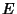anddenote subsets of. Further, let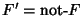be the complement of, so that(2)

Then the setcan be written as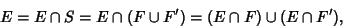(3)

where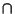denotes the intersection. Then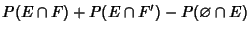(4)

whereis the Empty Set.

Letdenote the Conditional Probability ofgiven thathas already occurred, then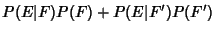(5)(6)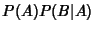(7)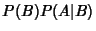(8)(9)(10)

A very important result states that(11)

which can be generalized to(12)

See also Bayes' Formula, Conditional Probability, Distribution, Distribution Function, Likelihood, Probability Axioms, Probability Function, Probability Inequality, Statistics